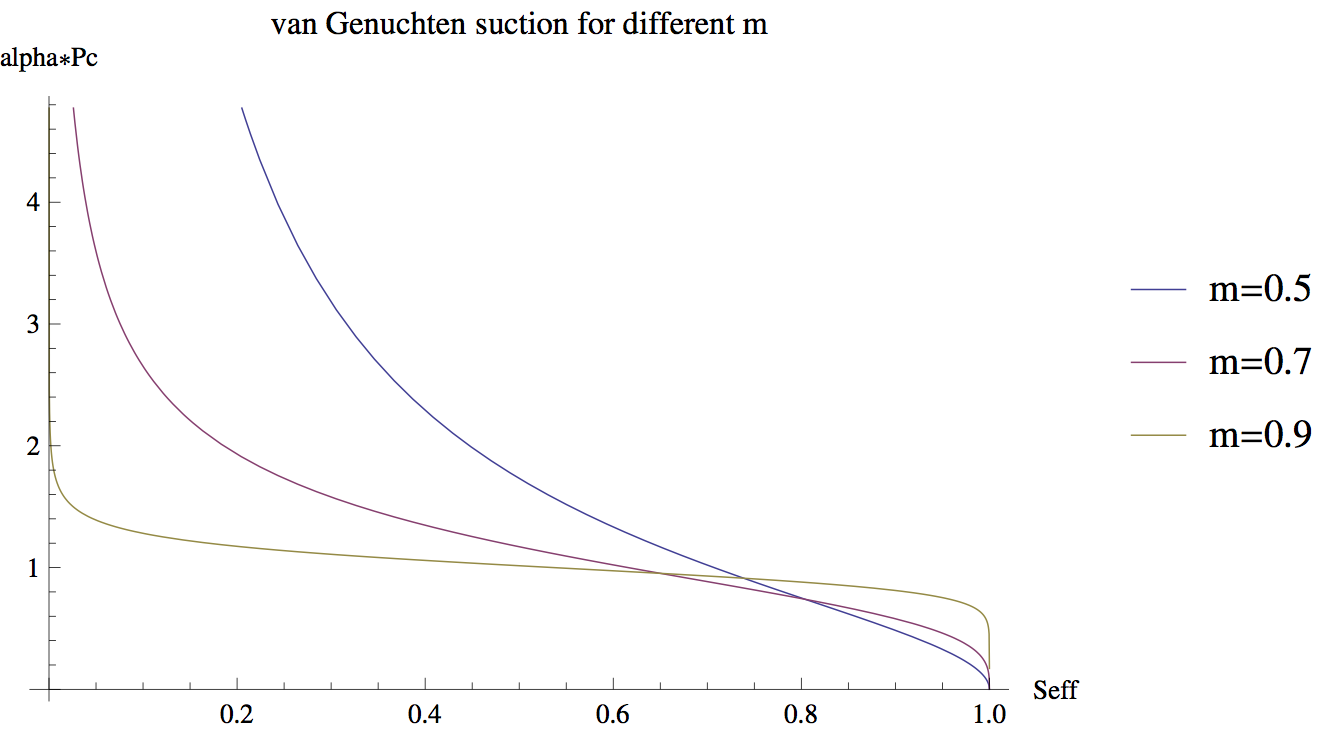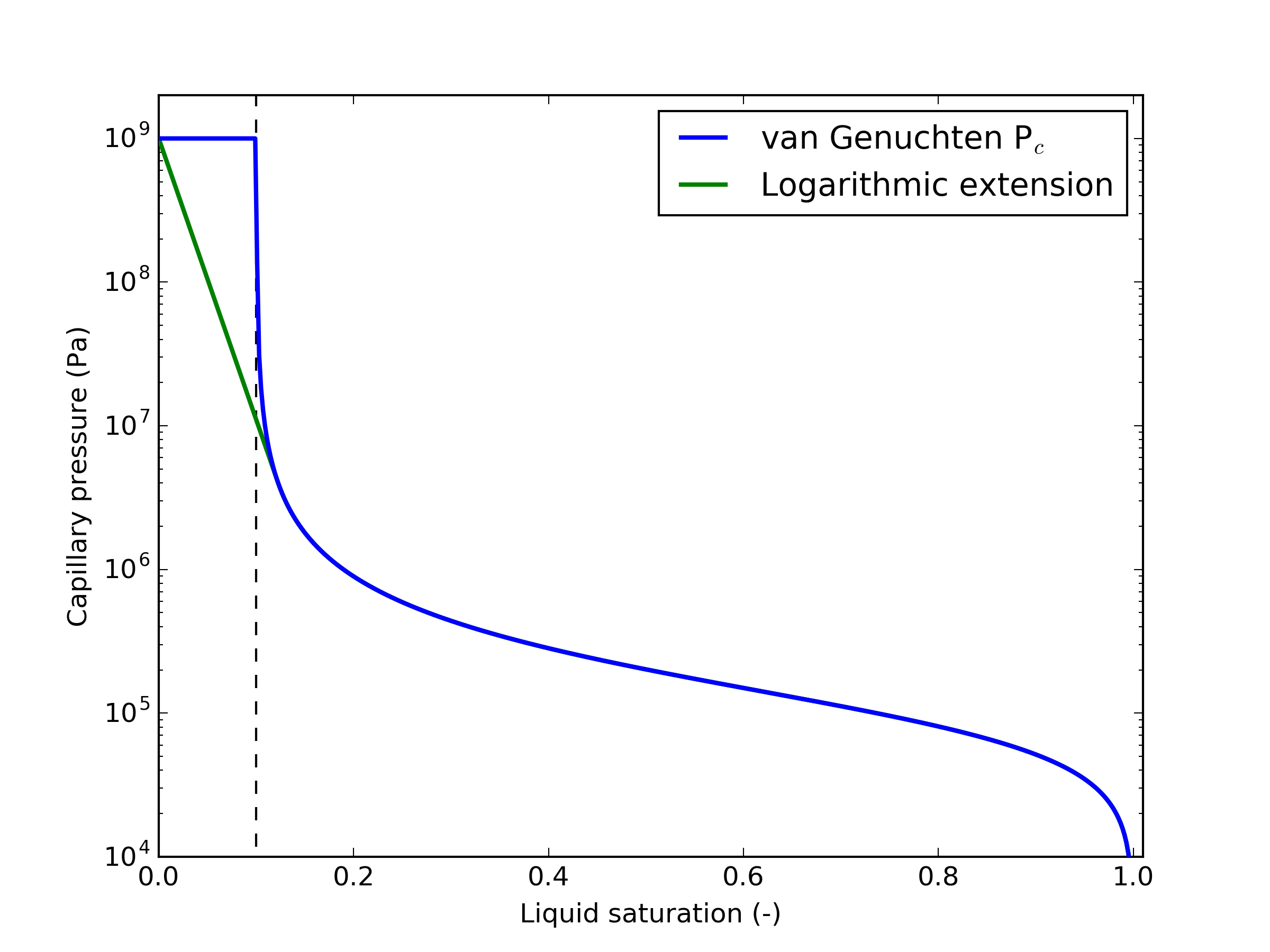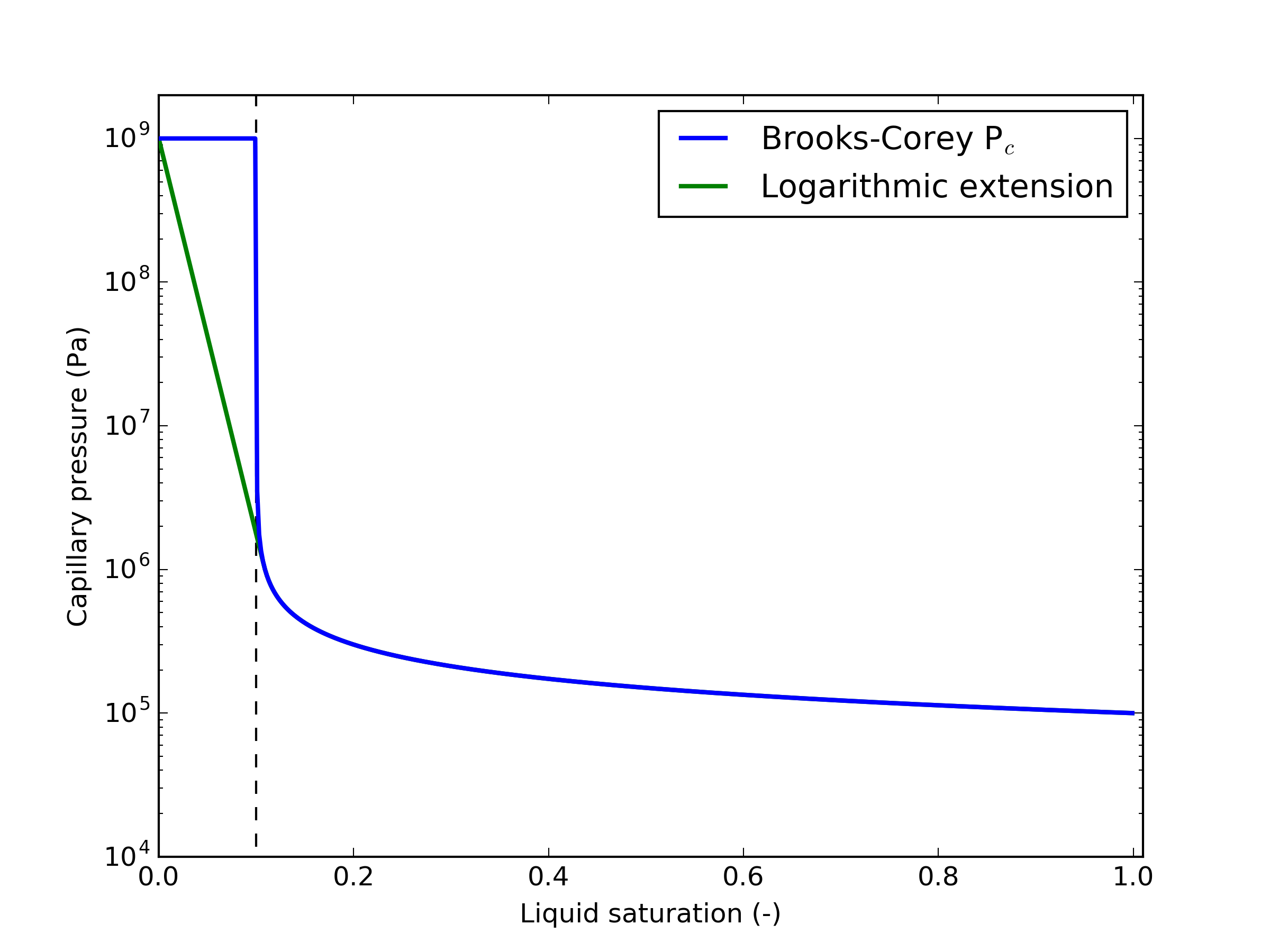# Capillary pressure

The capillary pressure is the pressure difference between two fluid phases in a porous medium that arises due to the interfacial tension between the fluid phases and the surface tension between fluids and the porous medium. Capillary pressure, , is commonly defined as (Bear, 1972) (1) where is the pressure of the non-wetting phase (typically the gas phase), and is the pressure of the wetting phase (typically the liquid phase). In the case of a single, unsaturated phase, it is common to use , which is the convention employed in PorousFlow. In this case, the capillary pressure is only relevant when , and this results in using the formulae below, otherwise, for , .

The capillary pressure is given by the Young-Laplace equation (Bear, 1972) (2) where is the interfacial tension, is the contact angle of the wetting phase on the surface of the porous medium, and is the radius of curvature at the interface, see Bear (1972).

Due to the difficulty in measuring and in real porous rocks, empirical and semi-impirical formulations for capillary pressure have been proposed that relate capillary pressure to effective saturation.

Several capillary pressure formulations are available in the Porous Flow module suitable for either porepressure formulations (where effective saturation and then saturation is calculated using capillary pressure), or porepressure-saturation formulations (where capillary pressure is calculated using the saturation).

In the following, the effective saturation is (3) This is the wetting-phase (liquid) effective saturation, and is the wetting phase saturation. The quantity is the wetting-phase residual saturation, and is termed sat_lr in MOOSE input files. The porepressure formulations compute as a function of capillary pressure, and hence which then appears in the fluid mass, the relative permeability, etc.

## Constant

PorousFlowCapillaryPressureConst

In this simple model, capillary pressure is constant (4) This formulation is useful for testing purposes.

## van Genuchten

PorousFlowCapillaryPressureVG

van Genuchten's capillary-pressure relationship is (Genuchten, 1980)

(5) or (6)

The effective saturation has been denoted by and is the porepressure, which is the negative of the capillary pressure: . Here and are user-defined parameters. The parameter must satisfy (7)

Sometimes the van Genuchten function is defined in terms of the parameter (8)

In van Genuchten's paper, he finds good fits with experimental data for various soils and rock when the parameter ranges between about 0.5 and 0.9 (meaning , roughly), and is between Pa and Pa. Figure 1 shows the shape of the van Genuchten suction, , as a function of .

Numerically there are three important features of Eq. (5):

• is a monotonically decreasing function of , which is necessary for a unique solution.

• as . As mentioned above, this is not justifiable physically, but numerically it is extremely advantageous over , as this latter version often causes algorithms to get stuck around . As also mentioned above, because of the low relative permeability around , physically realistic problems rarely explore the region.

• and as , for all . This ensures that there is continuity in the porepressure, , and the derivative around full saturation (remember that by definition for ). Also as if .

note

Users are encouraged to set m > 0.5Figure 1: Three values of are shown: 0.5, 0.7 and 0.9.

A modification of Eq. (5) is available where is replaced by and by . Here is a scale factor. This means the derivative as . Occasionally setting is advantageous in fully-saturated 2-phase simulations that have the two porepressures as their independent nonlinear variables.

## Brooks-Corey

PorousFlowCapillaryPressureBC

The Brooks-Corey capillary-pressure relationship is (Brooks and Corey, 1966)

(9) or (10) where is the threshold entry pressure, and is a user-defined exponent. Brooks and Corey originally related the exponent to the statistical distribution of pore sizes in the porous medium. A value of less than 2 was suggested for narrow distributions of pore sizes, while a value of greater than 2 was suggested for broad distributions of pore sizes.

## Broadbridge-White

PorousFlowCapillaryPressureBW

The Broadbridge-White capillarity relationship valid for small is (Broadbridge and White, 1988) (11) where (12) and is the Lambert W-function that satisfies . This is of limited use in real simulations, and is only used in the Porous Flow module for comparison with the analytical solutions of Broadbridge and White (1988) and Warrick et al. (1990) for multi-phase infiltration and drainage problems.

note

Only effective saturation as a function of capillary pressure is available in PorousFlowCapillaryPressureBW

## Rogers-Stallybrass-Clements

PorousFlowCapillaryPressureRSC

The Rogers-Stallybrass-Clements capillary relationship is (Rogers et al., 1983) (13) when the oil viscosity is exactly twice the water viscosity. This is of limited use in real simulations, and is only used in the Porous Flow module for comparison with the analytical solutions offered by the authors for multi-phase infiltration and drainage problems.

note

Only effective saturation as a function of capillary pressure is available in PorousFlowCapillaryPressureBW

## Logarithmic extension at low liquid saturations

Several of the capillary pressure formulations have capillary pressure and its derivative approach infinity while the liquid effective saturation approaches zero. While this is desirable for calculation of effective saturation as a function of capillary pressure, it is undesirable when calculating the capillary pressure numerically using saturation, often leading to numerical convergence issues.

By default, the numerical implementations of the capillary pressure curves implement a hard maximum when the effective saturation decreases below 0 in order to avoid unphysical values of capillary pressure and its derivatives. While this approach does avoid infinite values, it can lead to numerical difficulties for saturations close to residual (effective saturation close to zero) due to the discontinuous derivative of capillary pressure with respect to saturation.

To overcome this, the logarithmic extension detailed by Webb (2000) is implemented for low saturations in formulations where capillary pressure approaches infinity for small liquid saturations. An extension to the raw capillary pressure curve (14) is used for saturations less than a value . The value of is calculated so that the capillary pressure curve is continuous and smooth up to the maximum capillary pressure , see Figure 2 for an example for the van Genuchten capillary pressure, and Figure 3 for the Brooks-Corey capillary pressure. For these figures, , and (VG) or (BC).Figure 2: Logarithmic extension to van Genuchten capillary pressure curve below residual saturation.Figure 3: Logarithmic extension to Brooks-Corey capillary pressure curve below residual saturation.

1. J. Bear. Dynamics of fluids in porous media. Elsevier, New York, 1972.[BibTeX]
2. P. Broadbridge and I. White. Constant rate rainfall infiltration: a versatile nonlinear model, 1. analytical solution. Water Resources Research, 24:145–154, 1988.[BibTeX]
3. R. H. Brooks and A. T. Corey. Properties of porous media affecting fluid flow. J. Irrig. Drain. Div., 92:61–88, 1966.[BibTeX]
4. C. Rogers, M. P. Stallybrass, and D. L. Clements. On two phase filtration under gravity and with boundary infiltration: application of a Backlund transformation. Nonlinear Analysis, Theory, Methods and Applications, 7:785–799, 1983.[BibTeX]
5. M. Th. van Genuchten. A closed for equation for predicting the hydraulic conductivity of unsaturated soils. Soil Sci. Soc., 44:892–898, 1980.[BibTeX]
6. A. W. Warrick, D. O. Lomen, and A. Islas. An analytical solution to Richards' Equation for a Draining Soil Profile. Water Resources Research, 26:253–258, 1990.[BibTeX]
7. S. W. Webb. A simple extension of two-phase characteristic curves to include the dry region. Water Res. Research, 36:1425–1430, 2000.[BibTeX]